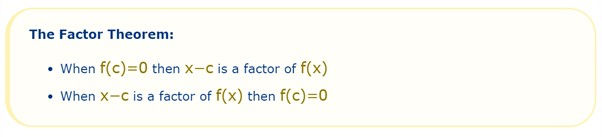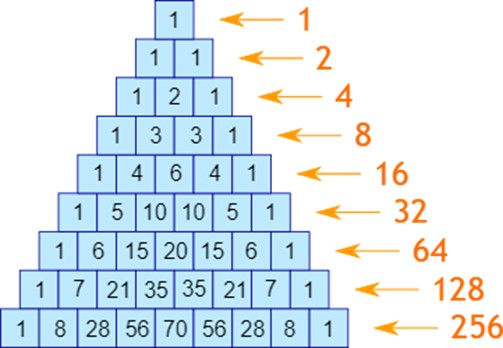top of page
Search

# The Year 11 Course – Why It’s Important and What You Need to Know

Why have I decided to dedicate an entire article to the year 11 course you ask? Well, it’s because as someone who sat the HSC last year, I saw first-hand just how examinable the year 11 topics are!

As you would know, ext.1 mathematics is one of the few subjects where the year 11 course content is explicitly tested in the HSC (not just assumed knowledge needed to answer some questions). And so it’s of the utmost importance that you don’t overlook this section of the course in your revision.

DID YOU KNOW?

Last year’s HSC exam consisted of over 50% of the marks going to year 11 topics??!

Now it can be argued that the year 11 course is in some ways more difficult than year 12 – it includes topics such as combinatorics, graphing and polynomials – all of which are some of the more challenging concepts in the maths ext. 1 course. In this blog I’ll be giving a brief rundown of these topics and some tricks when answering questions.

Topic 1: Polynomials

This topic involves:

• Polynomial division (essentially a form of long division)

• The factor theorem

• The remainder theorem

• Relationship between roots and coefficients

• Multiple roots

Key points to remember:

• The degree of the polynomial is determined by the power of the leading term (the highest power of the pronumeral)

• The degree of the polynomial tells you how many roots it can have (e.g. degree 5 means it has 5 roots), keeping in mind these could be multiple roots (e.g. a double root)

• The polynomial can be written as the divisor x the quotient + remainder (after doing polynomial division). This can be written as P(x) = divisor (what you were dividing by) times the quotient (the answer to the division) plus the remainder• Remainder theorem: if a polynomial P(x) is divided by (x – a) and the remainder R is a constant, then R = P(a). This means if I sub a into the polynomial, I will be left with the value of R. (remember to rearrange the divisor into the form (x – a).• Factor theorem: if a polynomial P(x) can be divided by (x – a) with a remainder of 0, then (x – a) is a factor of P(x) and P(a) = 0.Topic 2: Graphing

Topic breakdown and how I like to do it:

• Reciprocal functions (i.e. graphing 1/f(x), where f(x) is some function)

o Begin by drawing a vertical asymptote down any x-intercepts of the graph f(x) (this is because 1/f(x) cannot exist when f(x), the denominator, equals 0)

o Re-label the y-intercept as 1/y-intercept (e.g. 2 will become ½)

o Think about what the graph approaches on either side of the asymptote – you will essentially form a hyperbola shape with linear functions and a flat parabola shape between two vertical asymptotes (as f(x) à ∞, 1/f(x) à 0 and vice versa)

o Label any known points when drawing the graph (e.g. 1/1 = 1, so the point 1 stays the same)

• Square root functions

o Remember these graphs do not exist where f(x) is < 0 (you can ignore this part of the function/anything below the x-axis)

o Keep the x-intercept the same

o The square root of 0 is 0 and the square root of 1 is 1 (these points stay the same)

o Between 0 and 1, the graph will be slightly above the original graph

o Beyond 1 the graph will increase much less steeply

o If asked to sketch y2 = f(x), remember to reflect the graph in the x-axis as this means y = ±f(x)

o Note: remember to put a dot at 0 even if the graph becomes discontinuous as a square root graph exists at 0.

• Absolute value functions

o These graphs have a sharp point and often have a V shape

o Remember absolute value signs will make any negative values positive

o Reflect the negative part of the graph in the x-axis, keeping the sharp point in the middle

o For y = |f(x)|, reflect everything below the x-axis above

o For y = f(|x|), reflect everything on the right of the y-axis to the left

o For y = |f(|x|)|, first reflect everything below the x-axis above, and then reflect anything on the right of the y-axis to the left

Topic 3: Combinatorics

This topic involves:

• The fundamental counting principle

• Pigeonhole principle

• Permutations

• Combinations

• Counting techniques in probability

• Pascal’s triangle

Key points to remember:

• Combinatorics is undoubtably one of the most difficult topics in extension 1 maths with questions ranging in difficulty from simple to complex

• The pigeonhole principle states that if n items are put into m container s, with n>m, then at least one container must contain more than one item.• Permutations are used for ordered selections/arrangements (e.g. words, numbers, etc.); this can include arranging with restrictions or in a circle (remember to use (n – 1)! and to divide by the no. of repeated items when some elements are identical)

• Combinations are used when order is not important/a selection (e.g. committees, selections, groups, etc.)

• Pascal’s triangle can be used to find the coefficient of terms in the expansion of a binomial and is as follows:• Perms and combs can be used for probability problems involving real-world applications such as a deck of cards.

• If you are still struggling with this topic, below is a very useful link to the Khan Academy unit with video tutorials and practice questions I highly recommend for consolidating understanding and some extra practice:

All in all, don’t forget to revise your year 11 content! I hope these tips have been helpful!

Afrina